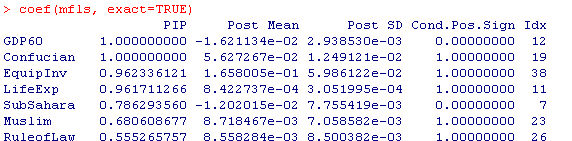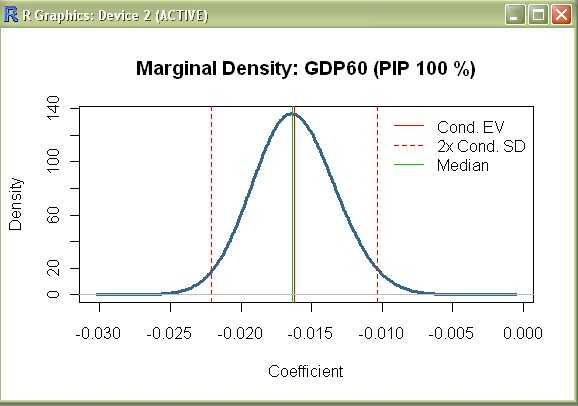# Using BMS to reproduce the results of Fernández, Ley and Steel (2001)

In their paper Model uncertainty in cross-country growth regressions (Journal of Applied Econometrics 2001), Fernández, Ley and Steel (FLS) demonstrate the use of Bayesian Model Averaging (BMA) for a cross-section economic growth data set. This tutorial shows how to reproduce the results with the R-package BMS.
A video tutorial (09:56) summarizes the essential part of this web tutorial.

## Getting R and BMS

Before continuing with the tutorial, you should have R installed (any version > 2.5) and the library BMS installed.

## How FLS Did It

The following is a short outline of how FLS set up the BMA routine in their cross-country growth paper:

• FLS use their own Fortran code to estimate the results in their paper. As described on p.6 they use their own dataset with N=72 observations and K=41 regressors.
• For Zellner's g-prior they use `g=max(N,K^2)` (p.7): please note that in the FLS nomenclature, their `g*` is always smaller than one. Our g follows the more frequently used nomenclature and corresponds to `g=1/g*` where `g*` is the parameter used in FLS.
• Their model prior is the uniform model prior (same prior probability for all models) - (p.7).
• For sampling through the model space, they use a 'Birth/Death' Metropolis MCMC (p.5) algorithm that wanders through model space by adding or dropping regressors from the current model. FLS use 1 million burn-ins and 2 million draws (p.7).
• They base their final posterior model probabilities on the 'exact' marginal likelihoods of an unidentified number of models (in their original Fortran code, 5000)

## Replicating: Demonstration

In order to demonstrate how to achieve the results, we will opt for a small scale version of the above that should take about a minute on a modern PC:

Leave the prior specifications the same, but take 100 000 burn-ins, then 200 000 draws with the same sampler, and save the best 2 000 models.

First, open R and load the package BMS with the following command

`library(BMS)`

Luckily, the FLS data set is already built in the BMS package: Get it with the command:

`data(datafls)`

The variable `datafls` is now a `data.frame` containing the FLS data, with 72 observations and 42 rows (the dependent plus the regressors).
Now we can sample our chain with:

`mfls = bms(datafls, burn=100000, iter=200000, g="BRIC", mprior="uniform", nmodel=2000, mcmc="bd", user.int=FALSE)`

Here, `bms` is the BMA sampling function (for more information type `?bms`). `datafls` is the data to be sampled through, where the first column is the dependent variable. `burn=100000` means 100 000 burn-in draws, with `iter=200000` MCMC iterations after that.
`g="BRIC"` sets the g-prior equal to max(N,K^2), something that was named 'BRIC' by subsequent authors as it bridges BIC and RIC. So in our case, this choice will set g=41^2. `mprior="uniform"` selects of course the uniform model prior (p(M)=K^-2), and `mcmc="bd"` selects the Birth/Death Metropolis sampler to iterate through the model space.

The sampler will loop through the model space and save aggregate statistics. In addition, the argument `nmodel=2000` forces it to store separately the best 2 000 models it encounters.
By the way: `user.int=FALSE` just hides output from the user (you), as this tutorial should not confuse you more than necessary.

## Results

For some basic information, you could use the command `summary(mfls)` to see how the sampler performed. But we are interested in Posterior Inclusion Probabilities (PIPs) and coefficients here. The command `coef.bma` will provide these:

`coef(mfls,exact=TRUE)`

The parameter `exact=TRUE` states that the PIPs and coefficient statistics should be computed on the basis of the best 2000 models saved in `mfls`. When comparing the results to the table on p.9 in FLS, one sees that the ranking and scale of PIPs pretty much matches up to FLS.

The above command returns a result comparable to the image below (click on it to get the full result):The column `PIP` details the posterior inclusion probabilities that corresponds to the table on p.9 of FLS. The column `Post Mean` shows the (unconditional) expected values (for information on the other columns, type `?coef.bma`).

However, there are some non-negligible differences in the exact numbers: The PIPs of the less important variables are smaller, the ones of the more important ones higher than in FLS. Let's see whether the coefficients based on aggregate statistics are better: type

`coef(mfls)`

This does `exact=FALSE` by default: This means that not marginal likelihoods are used for BMA, but the MCMC frequencies of the Metropolis sampler. Of course, more 'bad' models are included in the aggregate information than just in the 2000 best models.
When looking at the results, the differences to FLS flip now: Now the small PIPs are greater than in FLS, and the large PIPs are smaller than the ones in FLS (since we include more 'bad' models).

Another indicator is that the differences between MCMC frequencies and likelihoods seem not to be too large, but still not perfectly small. Type

`plotConv(mfls)`

to compare the Posterior model probabilities based on MCMC frequencies and likelihoods for the best 2000 models (see result)
Alternatively you could have tried

`cor(pmp.bma(mfls))`

which returns a correlation between MCMC frequencies and likelihoods of around 0.944 (`pmp.bma` prints out the two different concepts for the best 2000 models.)

## Even More Results

The analysis above show that our dry run is not perfect: on should try closer to FLS, with more iterations and saving more models, then the result should be closer too:

mfls2 = bms(datafls, burn=1e7, iter=2e7, g="BRIC", mprior="uniform", nmodel=5000, mcmc="bd")

This code should do better. Beware of executing it immediately, as it could take some time (probably more than 10 minutes).

Moreover, let's try to reproduce the posterior distribution of coefficients as on p. 10 of FLS:

`density(mfls,"GDP60")`

produces the first chart, namely the posterior density of the coefficient for initial GDP (cf. p. 10 in FLS):Note that the vertical bars in FLS are used to indicate results from Sala-i-Martin (AER 1997), and do not correspond to vertical bars here. (to suppress the vertical lines, use the optional argument `addons=""` as below).

`density(mfls,reg="Abslat",addons="",xlim=c(-.0004,.0004))`

The above command produces a chart that follows the characteristic shape of 'Absolute Latitude'. Similarly we can reproduce the chart for 'Fraction Catholic' with:

`density(mfls,reg="Catholic",addons="ml")`

There are several other functions in the BMS package that can be used to explore the data further: try out the following commands:

• `image(mfls[1:500])` plots the signs of the best 500 models (see result)
• `plotModelsize(mfls)` plot the prior and posterior model size distribution (see result)
• `beta.draws.bma(mfls[1:5])` produces the posterior expected coefficient values for the best 5 models (see result)
• `?c.bma` shows how to parallelize MCMC chains for larger tasks

## How to get Data from and to Excel

Above, we used the command `data(datafls)` to obtain the growth data that was already 'built-in' into the BMS package.
Of course the more 'typical' situation is to get data from an external file: and for Windows users, that's frequently Excel.
The following describes how to get data out of an Excel file under a Windows environment:

1. Open the Excel file datafls.xls
2. Select the cells you want to transfer to R (for the entire FLS data set, use the range from cell "A1" to "AP73")
3. Right-click and select "Copy..." (or press Ctrl+C)
4. Now in R, type the following:
`datafls=read.table("clipboard-128", sep="\t", header=TRUE)`
This means that R should read a data.frame from the clipboard, with the typical Excel/Windows column separator (the tabulator) and that the first row contains the column names
5. You are done: type `datafls` to verify

Equivalently, one might copy the coefficient results to Excel with the following commands:

```mcoef = coef(mfls, exact=TRUE)
write.table(mcoef,"clipboard-128",sep="\t")```

Right-click somewhere in an Excel cell and select 'Paste'

Similarly, for copying charts , it suffices to right-click them and select 'Copy as bitmap' (under Windows) to paste them to another application. For plotting charts directly to file, consider the example in `help(png)`